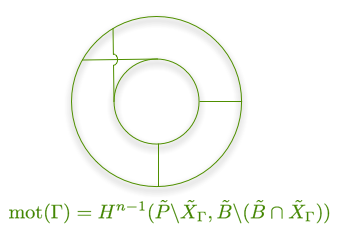# Online seminar on motives and period integrals in Quantum field theory and String theory### Organizers :Federico Zerbini (IPhT CEA-Saclay), Pierre Vanhove (IPhT CEA-Saclay)

Talks (titles, abstracts and slides) for the years 2017 - 2019 -- 2020 -- 2021 -- 2022 -- next talk

This is the page for the online seminar on motives and period integrals in Quantum field theory and String theory. The seminars will solely take place online. The Zoom Meeting ID and the password, will be sent to the participants by email before the semaine via the mailing list

If you are interested in joigning this seminar series you should contact the organisers.

## 2022

Time: 11h00 (Paris, France Time)
Bonn University

TBA

## 15 June 2022

Time: 11h00 (Paris, France Time)
##### Pierre Vanhove
IPhT CEA-Saclay

Analytic structure of cosmological correlators

We study the analytic structure of late-time cosmological correlations functions in rigid de Sitter space time. It was recently pointed out that the cosmological correlators can be mapped to Euclidean anti de Sitter correlators, therefore reducing the computation to correlator in EAdS. For the case of conformally coupled scalars we show that the evaluation of the AdS Witten diagrams can be reintepreted as flat-space Feynman integrals. This simplifies drastically the perturbative computations, and allow to analytical evaluations of the correlator in perturbation. From these analytical evaluations, we extract loop corrections to the anomalous dimensions, and get some insights into the analytic structure of conformal correlators in rigid AdS and dS.

## 11 May 2022

Time: 11h00 (Paris, France Time)
##### Daniele Dorigoni
Durham University

Poincaré series for Modular Graph Forms and Iterated integrals

pdf de l'exposé

I will present Poincaré series representations for infinite families of modular forms including depth-two non-holomorphic modular graph forms as they appear in the low-energy expansion of closed-string scattering amplitudes at genus one. These Poincaré series are constructed from iterated integrals over holomorphic Eisenstein series and their complex conjugates, decorated by suitable combinations of zeta values. The space of modular forms thus obtained is an extension of the world of modular graph forms, and it does also contain iterated integrals of holomorphic cusp forms and their L-values.

## 27 April 2022

Time: 11h00 (Paris, France Time)
##### Hans Jockers
Mainz University

The Limiting Value of D-brane Superpotentials

pdf de l'exposé

Mirror symmetry predicts the "classical" algebraic and transcendental invariants of degenerate Calabi-Yau threefold to match with the symplectic "quantum" invariants of the mirror manifold. An instance of this correspondence arises in the context of open-string mirror symmetry, in which the Abel-Jacobi map of families of algebraic cycles corresponds to instanton-corrected invariants of Lagrangian submanifolds of the mirror manifold. Using a technique introduced by Griffiths, Green and Kerr, we calculate certain values of the Abel-Jacobi map for specific algebraic cycles in a suitable degeneration limit of the Calabi-Yau threefold, recovering previously obtained numerical results. We interpret the calculated invariants in the context of open-string mirror symmetry. This talk is based on work in progress with Dave Morrison and Johannes Walcher.

## 16 March 2022

Unusual time Time: 16h00 (Paris, France Time)
##### Simon Caron-Huot
Mc Gill University

Causality and modifications to Einstein's gravity

pdf de l'exposé

Does our world respect causality at all energy scales? We explore constraints on gravitational dynamics which stem from this assumption. Parameterizing long-distance effects of possible new heavy particles using effective field theory (EFT), we investigate causality of 2 to 2 scattering processes. Due to its growth with energy, the gravitational force turns out to be particularly constrained. I will present two-sided bounds which show that a wide class of modifications to four-dimensional Einstein's gravity, require either the existence of light higher-spin states, or violation of causality as we understand it.

## 23 February 2022

Time: 11h00 (Paris, France Time)
##### Michael B. Green
Cambridge Univ. and QMUL

Exact expressions for integrated correlators in $\mathcal{N}=4$ supersymmetric Yang--Mills

pdf de l'exposé

This talk will present the exact expression for an integrated correlator of four BPS operators in ${\cal N}=4$ supersymmetric Yang-Mills with any classical gauge group $G_N$. I will show that this can be expressed as a two-dimensional lattice sum, which is a function of $N$ and of the complex Yang--Mills coupling, $\tau=\theta/2\pi + i 4\pi/g_{_{YM}}^2,$. This expression is manifestly invariant under $SL(2,Z)$ duality in the case of simply-laced gauge groups ($SU(N)$ and $SO(2N)$), and $\Gamma_0(2)$ in the case of non-simply laced gauge groups ($SO(2N+1)$ and $USp(2N)$). Furthermore, it satisfies a striking `Laplace difference' equation that relates the correlator for gauge group $G_N$ to those for gauge groups $G_{N-1}$ and $G_{N+1}$. For any $G_N$ the correlator can be expressed as an infinite rational sum of non-holomorphic Eisenstein series with integer indices, and can be expanded to arbitrary order in perturbation theory, together with an infinite series of instanton terms. This reproduces and extends perturbative and non-perturbative ${\cal N}=4$ SYM results. In the $N\to \infty$ limit the expansion in powers of $1/N$ has coefficients that are rational sums of non-holomorphic Eisenstein series with half-integer indices and is holographically related to the low energy expansion of the type IIB superstring four-graviton amplitude in an orientifold of $AdS_5\times S^5$ if $G_N=SU(N)$, or in $AdS_5\times S^5/Z_2$ if $G_N=SO(2N)$, $SO(2N+1)$ or $USp(2N)$.

## 19 January 2022

Time: 11h00 (Paris, France Time)
##### Federico Zerbini
IPhT CEA-Saclay

Iterated integrals on Riemann surfaces, flat connections and polylogarithms

pdf de l'exposé

Iterated integrals encode important information about fundamental groups, and are a rich source of periods of mixed motives. Such integrals are often encountered in the computation of Feynman amplitudes. Motivated by string theory computations, I will focus on the case of Riemann surfaces, reviewing the known genus-zero and genus-one case, which give rise to classical and elliptic polylogarithms, and reporting on recent progress at higher genus in collaboration with Benjamin Enriquez. More specifically, we have constructed explicit flat connections, conjugated to higher-genus analogues of the KZ-connection, which give rise to spaces of multi-valued functions that may be viewed as higher-genus analogues of polylogarithms.